DigDB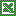Add-in Tools for Excel Elites
Who else wants to do a 10-hour Excel analysis in 15 minutes? (more ...)

Features | Testimonial | Download free 15-day trial | Purchase | FAQ | Contact

### Median - Roll-up (pivot table) with Medians

Excel has a median formula, but it does NOT support median in Pivot Table or Subtotal. Pivot table and subtotal only has the Average, a.k.a Mean.

The Median is the number in the middle of a set of numbers; that is, half the numbers have values that are greater than the median, and half have values that are less. If there is an even number of numbers in the set, then MEDIAN calculates the average of the two numbers in the middle. For example:

• MEDIAN of the set of numbers (1, 2, 3, 4, 5) equals 3
• MEDIAN of the set of numbers (1, 2, 3, 4, 5, 6) equals 3.5, the average of 3 and 4
Below is an Employee list table, suppose we want to find out the median employee 'Age' of each 'Department'.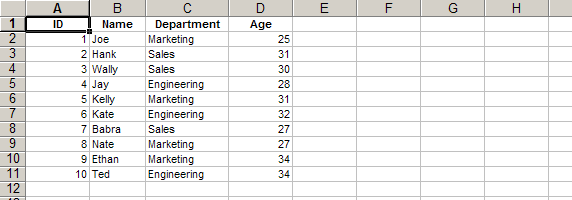Practice file - median-pivot-table-demo.xls (16k)

1. Click any cell within your list area, Invoke 'DigDB->Roll up...'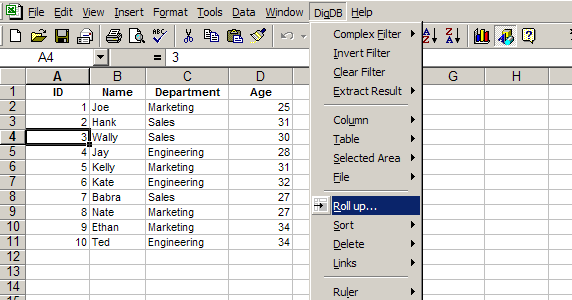2. Set summary criteria: find the middle (Median) Age value in each Department

• Use the Calculations area to select 'Median' of 'Age', use the upper 'Add' button to add to the criteria box
• Use the Group-by(s) area to select 'Department', then use the lower 'Add' button to add to the criteria box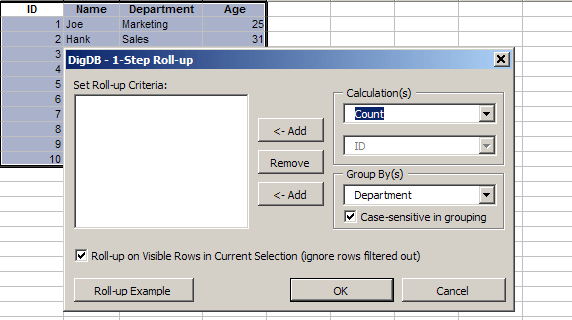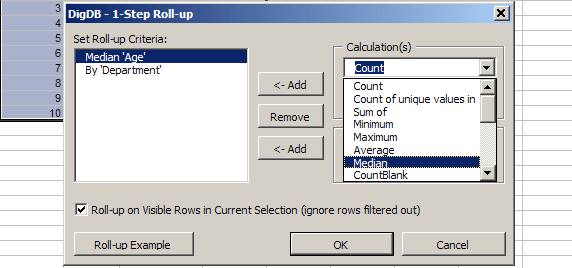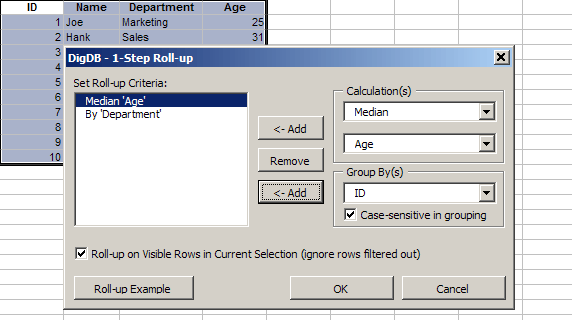3. Click 'OK' to get the roll up. Result is shown in a new list on a new sheet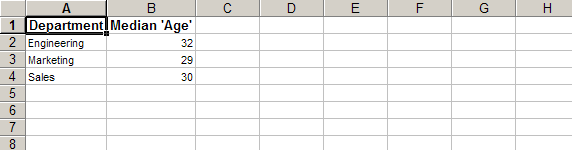Besides Median, DigDB's roll up also supports Count of Unique values, MODE, Standard Deviation and a total of 21 statistical functions. This give users far more aggregate power than Pivot table and Subtotal.

### Need more?

• Browse the complete list of DigDB's powerful features listed on the front page.
• If you see errors or things not working, check out our error reference page.

DigDBAdd-in Tools for Excel Elites